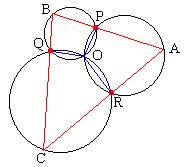# A Property of Concurrent Circles

The applet below, what is it about? A few things I am willing to tell you right away. First of all, there are several circles concurrent at a point. All circles can be changed by dragging their centers.

There is also a polygon inscribed into this collection of circles with one vertex on each circle. The vertex on the rightmost circle is chosen randomly (and will change its location when you press the Reset button), the rest are constructed by drawing the straight lines through the intersection of the circle. A remarkable thing happens - what is it?

1 October 2015, Created with GeoGebra

Hint

ProofCopyright © 1996-2018 Alexander Bogomolny

The sides of the polygon pass through the points of intersection of the circles. The diagram may be obtained in an alternative way as well. For definitiveness sake, count the circles counterclockwise starting with the rightmost. Select a point on the first circle. Draw a line from this point through the point of intersection of the first two circles other than the point common to all circles.) Extend the line until it intersects the second circle at some other point. From here, draw a line through the second intersection point, and so on until you come back to the fixed circle. What is interesting here is that the final and the initial points of the construction always coincide with each other.

The proof of this result is not difficult at all and uses some properties of angles inscribed into circles. We discussed these properties on several occasions:

A problem from the 2007 Irish Mathematical Olympiad is a pretty reformulation of the case of three concurrent circles (with an omission of one), that demonstrates how shifting a view point sheds extra light on a known fact.

Note another curious fact. When all circles have equal radii and their centers are evenly spaced on some circle. Then the resulting polygon is always regular regardless of the selection of the first vertex.Copyright © 1996-2018 Alexander BogomolnyI give a proof for three circles. Assume three circles have a common point O and also intersect pairwise at points P, Q, and R. A point A is selected on one of the circles. Then we draw the line AP and extend it to the intersection with another circle at the point B. Next BQ is extend past Q to BC and lastly CR is extended past R. I want to show that CR hits the line AB exactly at the point A.

Let it be some other point A' and consider ΔA'BC. In this triangle, angles B and C do not depend on the position of A. ∠B always subtends the same arc (POQ) and the same is true for ∠C. Therefore, the sum of angles B and C is constant and, since the sum of the angles in every triangle is the same, ∠A' is also constant.

From here we conclude that when A changes the locus of points A' is a circular arc that passes through the points P and R. To show that it actually coincides with the rightmost circle, we again count angles. Draw the lines OP, OQ, and OR. Obviously, ∠POQ + ∠B + 180°. By the same token, ∠QOR + ∠C = 180° and ∠ROP + ∠A = 180°. Also, of course, ∠POQ + QOR + ∠ROP = 360° from which it follows that ∠A = 180° - ∠A - ∠B. In other words, the angles A and A' are equal and therefore lie on the same circle. Thus the points A and A' coincide.

### Remark

Assume, as in the diagram, A is one of the vertices of the thus obtained polygon with points P and Q on adjacent sides. If A and P coincide, then circle APQ is tangent to the side AB in A.

### References

1. D. Wells, You are a Mathematician, John Wiley & Sons, 1995### Chasing Inscribed AnglesCopyright © 1996-2018 Alexander Bogomolny

 64860190